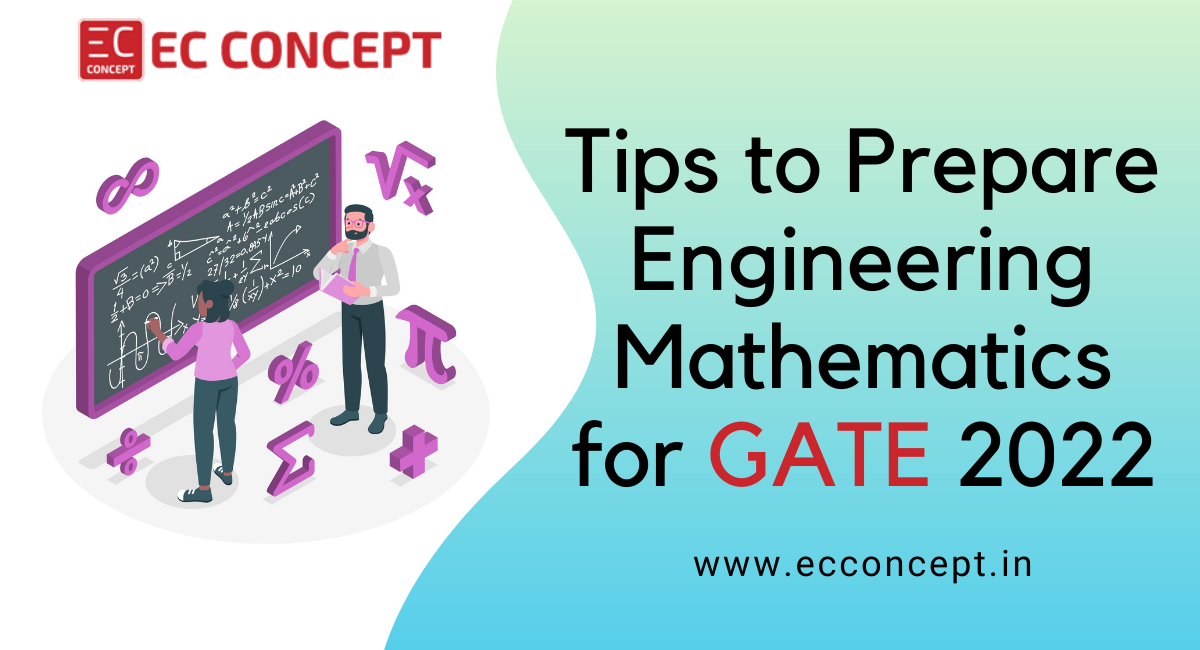### Recent News

• #### How To Score Under 100 Ranks In GATE 2022 Examination?

Sep 27,2021- GATE

• #### How can mock tests help you to ace the examination?

Aug 06,2021- Online Test Series# How To Prepare Engineering Mathematics For GATE 2022?

• 0

GATE (Graduate Aptitude Test in Engineering) is jointly organized by the IISc. (Indian Institute of Science), and the seven IITs (Indian Institute of Technology) to test the skills and competencies of engineering students aspiring to pursue Master and Doctoral programs with the Ministry of Education or avail Government scholarships. Engineering Mathematics holds a significant weightage and it has to be noted that 13 marks in the GATE examination are from Engineering Mathematics. It is a vast subject and preparing it at a stretch at the last moment is overwhelming and highly impossible. It requires strategizing and proper planning to pull out a good score.

This article aims to shed light on the ways to prepare Engineering Mathematics for the GATE examination in a structured manner within a short span of time.

## Cramming the subject before a week may not work

It is difficult to memorize word by word and may never work with Engineering Mathematics. It is only a waste of time and energy. Try to remember the formula, understand the concepts in every chapter, and ways to apply them to solve problems in real-time.

## You can create notes at the end of each chapter

Note making is an art and using color pens, bullets, and other creative means to craft an overall picture of the subject is the easiest strategy to follow during competitive exam preparation. This might help in recapping important concepts and revising the subjects before the exam. You can also consider creating a separate formula notebook.

## Take up more GATE mock tests and practice exams

Practice improves your speed when you take up tests in a real-time environment. Try to take as many GATE mock tests as possible in a simulated environment, and by doing so, you learn to manage your time effectively. This improves your scores ultimately as you learn to master the art of answering more questions in a short time and gain a competitive edge. You also get to understand your weakness post which you can work out ways to sort them out.## Study systematically and practice regularly

Work out a study plan. You may not be able to ace the Engineering Mathematics subject unless you practice and learn to apply formulas and concepts in real-time. Once you complete each chapter, practice the questions that were asked from that chapter in the previous year’s question papers. You can choose to attend online classes and webinars as per your routine and follow a systematic procedure to prepare for the GATE Exam. Such online courses come with the GATE test series using which you can test your knowledge periodically.

Listed below are the important concepts and preferences under each chapter that you may use while doing exam preparation for the Engineering Mathematics subject in GATE 2022.

1. Linear Algebra - Focus on Eigenvalues and vectors, rank & determinant of matrices, and linear system equations.

2. Calculus- Focus on maxima and minima in single variable calculus., limits, and gradient, divergence, curl, and integral theorems (Gauss’s, Green’s and Stoke’s Theorem) from vector calculus.

3. Differential Equations- Concentrate on first-order as well as higher-order differential equations. Also, remember some special equations like Bernoulli and Euler equations.

4. Probability and Statistics- Emphasize more on mastering Bayes Theorem as it will help you answer most of the questions. Due importance should be given to random variables like Poisson’s distribution, statistical concepts including mean, median, mode, coefficient of correlation, etc.

5. Numerical methods - Focus on solving equations using methods like Newton-Raphson and Bisection and numerical integration methods like Trapezoidal rule and Simpson’s rule.

6. Complex Analysis- Learn in detail the Cauchy-Riemann equations, Residue method of integration and Taylor series.